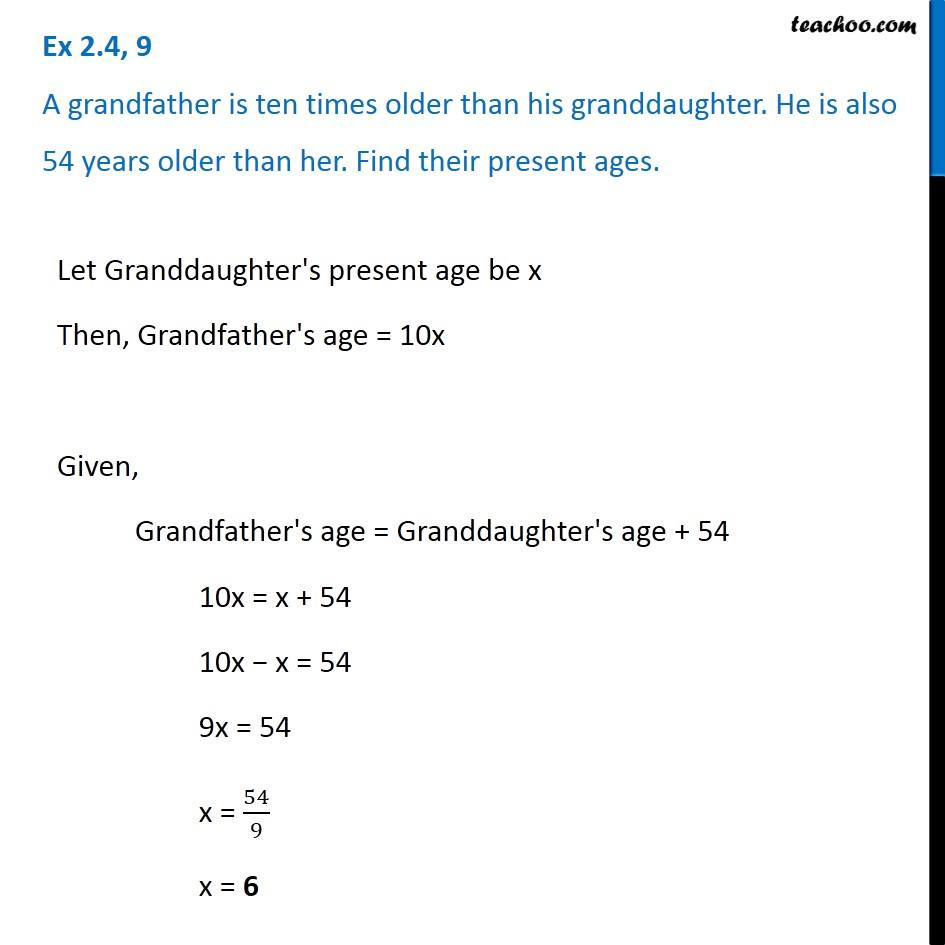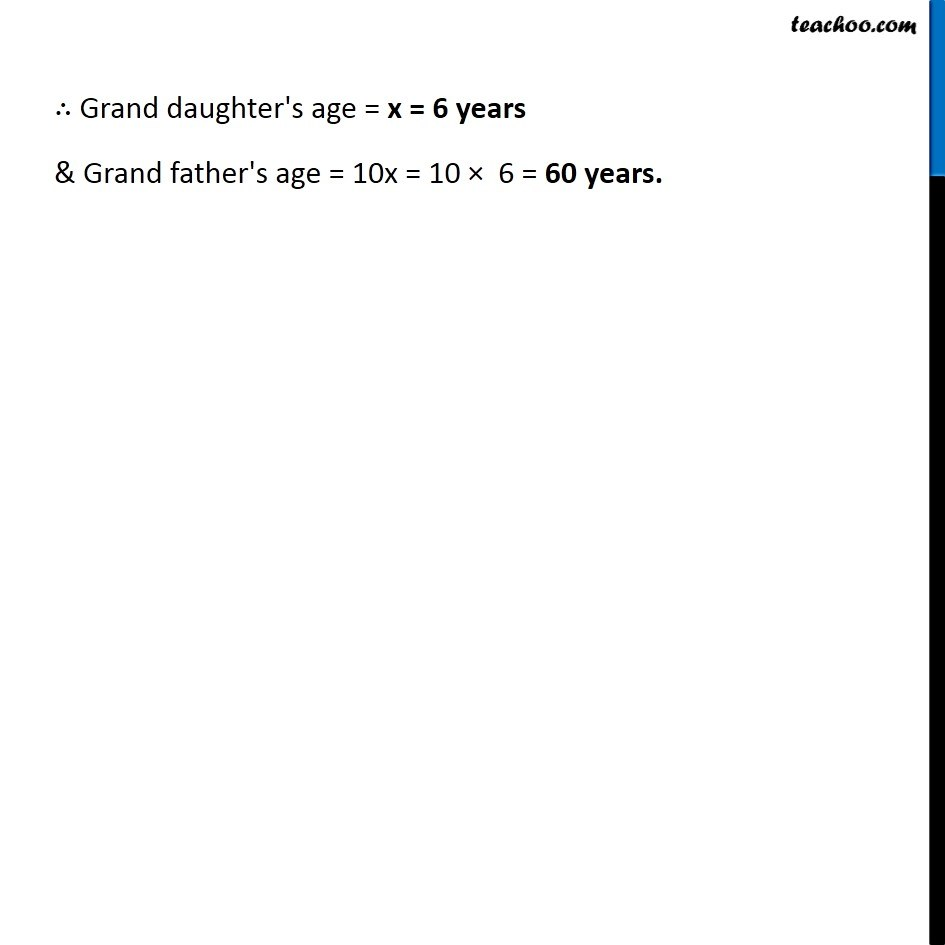Solving difficult equations

Chapter 2 Class 8 Linear Equations in One Variable
Serial order wiseLearn in your speed, with individual attention - Teachoo Maths 1-on-1 Class

### Transcript

Question 9 A grandfather is ten times older than his granddaughter. He is also 54 years older than her. Find their present ages.Let Granddaughter's present age be x Then, Grandfather's age = 10x Given, Grandfather's age = Granddaughter's age + 54 10x = x + 54 10x − x = 54 9x = 54 x = 54/9 x = 6 ∴ Grand daughter's age = x = 6 years & Grand father's age = 10x = 10 × 6 = 60 years.